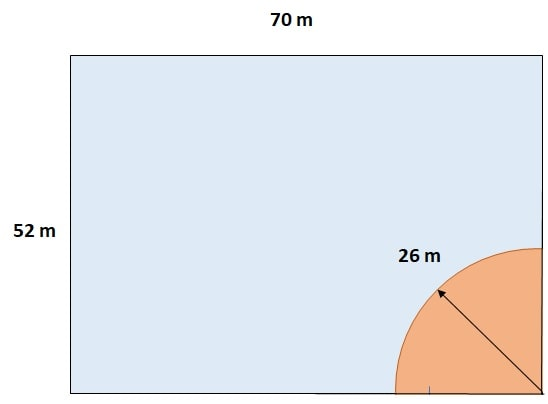Q) A horse is placed for grazing inside a rectangular field 70 m by 52 m and is tethered to one corner by a rope 21 m long. On how much area can it graze?

Ans: Let’s draw a diagram and plot the given information.

(Note: your question will become clearer and your answer will never be wrong if you draw a diagram)Since horse is pegged to a corner; it can only graze a quarter of a circle in the given field.

Hence, we have nothing to do with the dimensions of the field or its area. all we need is the circle made by horse inside the field. Here, since the horse is tied up by 21m long rope, hence the radius of the circle it will make is 21 m.

Next, we know that the area a quarter of a circle of radius r is given byFrom above diagram, area grazed by horse with 21m rope will be quarter of a 21 m radius circle.

Hence area grazed ====== 346.50 m2

Therefore, with 21m rope, grazed area by horse will be 346.50 m2 .

Note: if it was asked in the question, that what portion of the field area or what ratio of the field area will be grazed by horse, then dimensions of the field would have been important. In that case, we will take ratio of area grazed divided by field area.

Scroll to Top